Courses

# Semiconductor Diode - MSQ

## 10 Questions MCQ Test Topic wise Tests for IIT JAM Physics | Semiconductor Diode - MSQ

Description
This mock test of Semiconductor Diode - MSQ for Physics helps you for every Physics entrance exam. This contains 10 Multiple Choice Questions for Physics Semiconductor Diode - MSQ (mcq) to study with solutions a complete question bank. The solved questions answers in this Semiconductor Diode - MSQ quiz give you a good mix of easy questions and tough questions. Physics students definitely take this Semiconductor Diode - MSQ exercise for a better result in the exam. You can find other Semiconductor Diode - MSQ extra questions, long questions & short questions for Physics on EduRev as well by searching above.
*Multiple options can be correct
QUESTION: 1

### The built-in potential of p-n junction diode is a function of.

Solution:

Formation of potential of p-n junction diode depends on temperature, biased voltage and doping density.
The correct answers are: Temperature, Biased voltage, Doping density

*Multiple options can be correct
QUESTION: 2

### An ideal switch is one which has :

Solution:

Characteristics of any ideal switch is that it should offer zero resistance when ON and infinite resistance when OFF.
The correct answers are: Zero resistance when ON, Infinite resistance when OFF

*Multiple options can be correct
QUESTION: 3

### For a full wave rectifier:

Solution:

Full wave rectifier consists of two diodes each with different polarity ends which help it keep running both in positive and negative half. Average or dc value of load current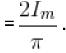The correct answers are: We need two diodes placed at opposite polarity, Ripple factor = 0.483, Maximum Rectification efficiency η = 81.1 %

*Multiple options can be correct
QUESTION: 4

Which of the following statements is true in case of zener diode?

Solution:

We know that zener diode has sharp breakdown voltage. It is always reverse biased and heavily doped diode. There is similarity between forward characteristic curve of a diode.
The correct answers are: A zener diode has sharp breakdown voltage, A zener diode is always reverse biased, The zener diode is a heavily doped diode

*Multiple options can be correct
QUESTION: 5

Consider the following statements regarding the magnitude of barrier potential of a p- n junction. Which of them are true?

Solution:

When a p-type semiconductor having holes as majority carrier is clamped with a ntype semiconductor having electron as majority carrier.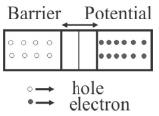Some electrons from n-side goes to p-side and some holes goes to n-side, thus creates a potential barrier, which
(i) depends on temperature
(ii) depends on fermi level
(iii) forbidden energy gap Eg
(iv) majority carriers
The correct answers are: It depends on difference between fermi levels on two sides of fermi level, It depends on forbidden energy-gap on two types of semiconductors, It depends on impurity concentration in p and n-type semiconductors

*Multiple options can be correct
QUESTION: 6

Which of the following devices is forward bias in its normal mode of operation.

Solution:

Zener diode and Avalanche photodiode operates only in reverse bias condition. The correct answers are: Solar cell, LASER diode

*Multiple options can be correct
QUESTION: 7

If the barrier potential change with biasing of a p-n junction diode, then the barrier potential will.

Solution:

For a p-n junction diode, barrier potential decreases with forward and increases with reverse biasing.
The correct answers are: increase with reverse bias, decrease with forward bias

*Multiple options can be correct
QUESTION: 8

For an intrinsic semiconductor,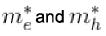are respectively, the effective masses of electrons and holes near the corresponding band edges. At a finite temperature, the position of fermi level:

Solution:

The finite temperature, the position of fermi level would depend on bothThe correct answers are: depends on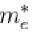, depends on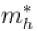*Multiple options can be correct
QUESTION: 9

Which of the following statements are true for a clamper?

Solution:

Any clamper consists of a diode, resistor and capacitor. It does not require any inductor. It does not change the appearance but only shifts the dc level of any given signal.
The correct answers are: does not change appearance of input signal, shifts dc level of the waveform

*Multiple options can be correct
QUESTION: 10

Which of the following electronic devices are used to make a clamper?

Solution:

A normal clamper consists of a diode, a resistor and a capacitor that shifts a waveform to a different dc level without changing the appearance of the applied signal.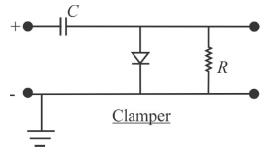The correct answers are: Capacitor, Diode, Resistor